## Horizontal Road on a Ideal Terrain

IDEAL TERRAIN - plane with a slope

NEUTRAL LINE or LINE OF TRANSITION - is the intersection of the subgrade and the terrain

 EMBANKMENT or FILL - is the plane or the part of a surface with the slope $$n_N=\frac{2}{3}$$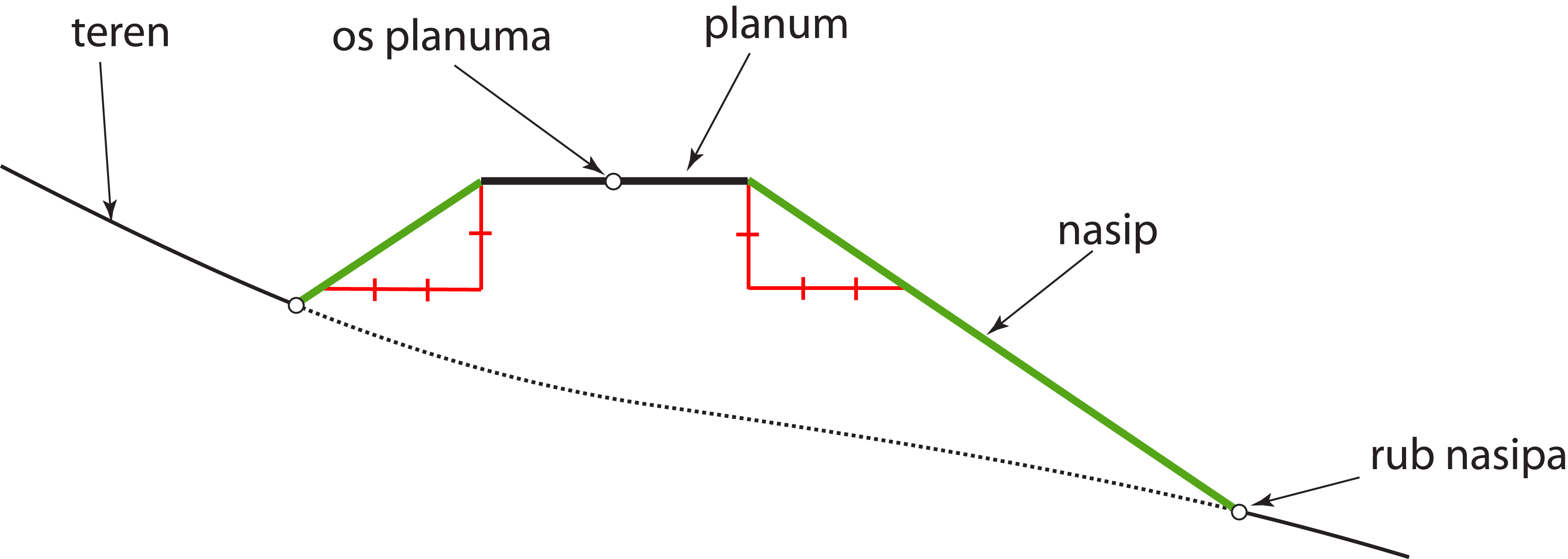EXCAVATION or CUT - is the plane or the part of a surface with the slope $$n_U=\frac{4}{5}$$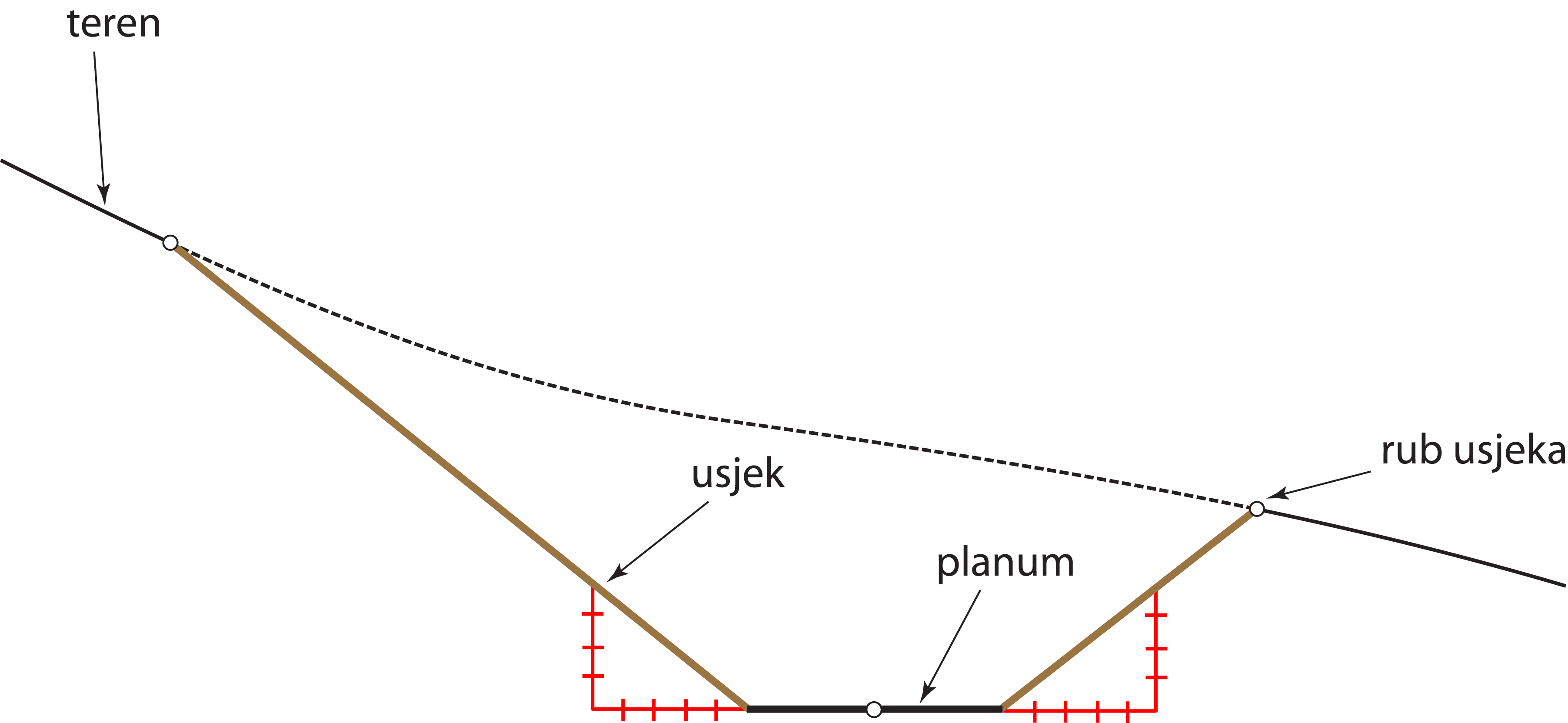### Straight horizontal road on a ideal terrain

Exercise 1: Let the plane $$\Sigma$$ be the ideal terrain with the slope of 20%. On the template this plane is given with the scale of slope and the horizontal with the elevation 0.
The straight horizontal road has the width 10m and the elevation 10. On the template the road is given with its axis.
Solve the earthwork for building this road.

1st solution - elevated projection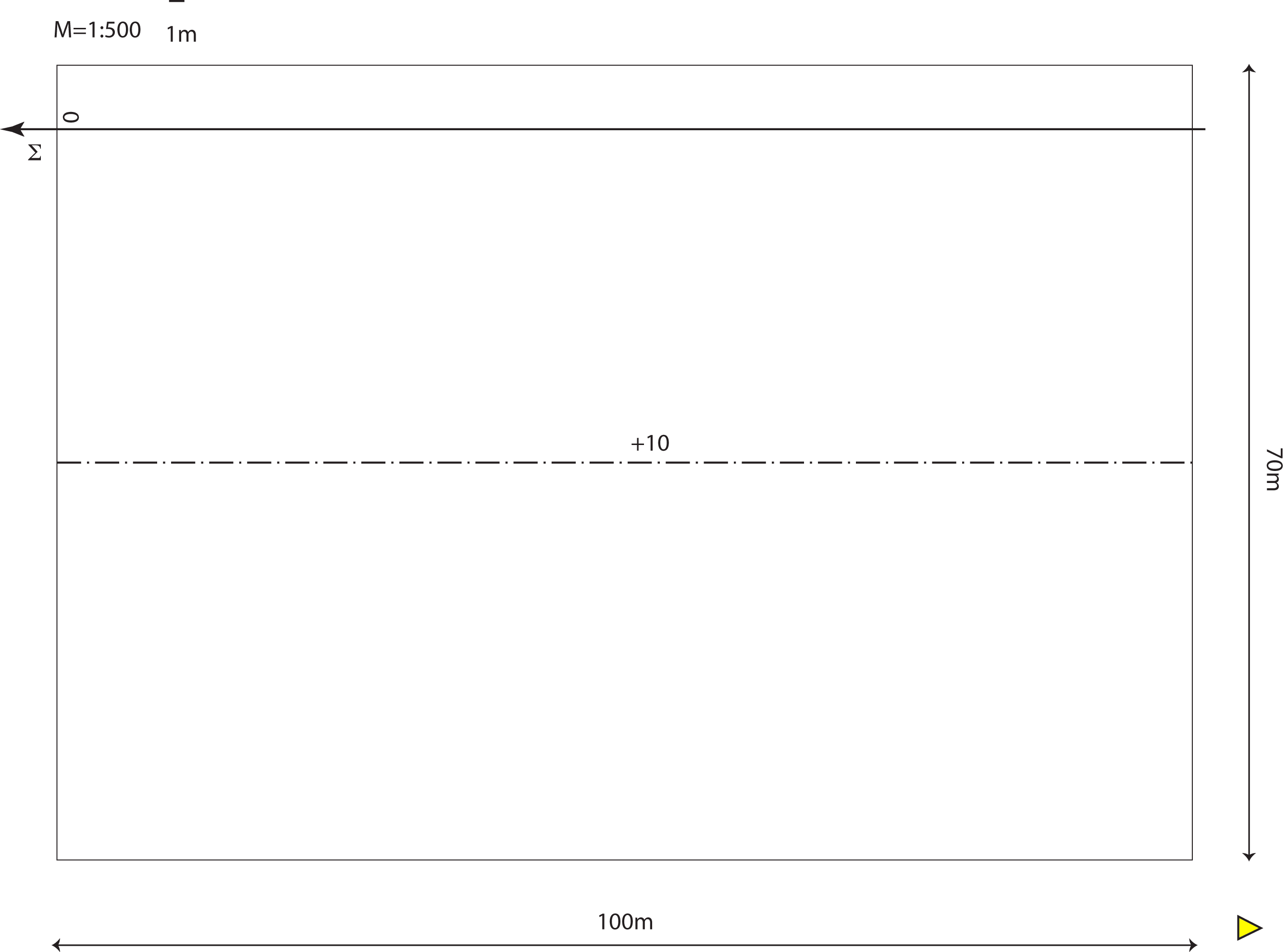Steps of the construction:
• Construct the horizontals of the plane $$\Sigma$$, $$i_\Sigma=5m$$.
• Construct the boundary line of the subgrade.
• Determine the neutral line.
• Find the scales of slope for the fills and construct the horizontals of those planes.
• Determine the boundary line between the ideal terrain and fills, i.e its intersection.
• Find the scales of slope for the cuts and construct the horizontals of those planes.
• Determine the boundary line between the ideal terrain and the cuts, i.e its intersection.

2nd solution - program Rhino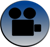ideal terrain, subgrade and neutral transitionfills (embankments)cuts (excavations)final image

### A horizontal road on a circular arc on a ideal terrain

EXERCISE 2: Let the plane $$\Sigma$$ be the ideal terrain with the slope 20%. On the template this plane is given with the scale of slopes and the horizontal with the elevation 0.
The road, having the wifth of 10m, streches along a circular arc which is on the template given with its axis.
Solve the earthwork for building this road.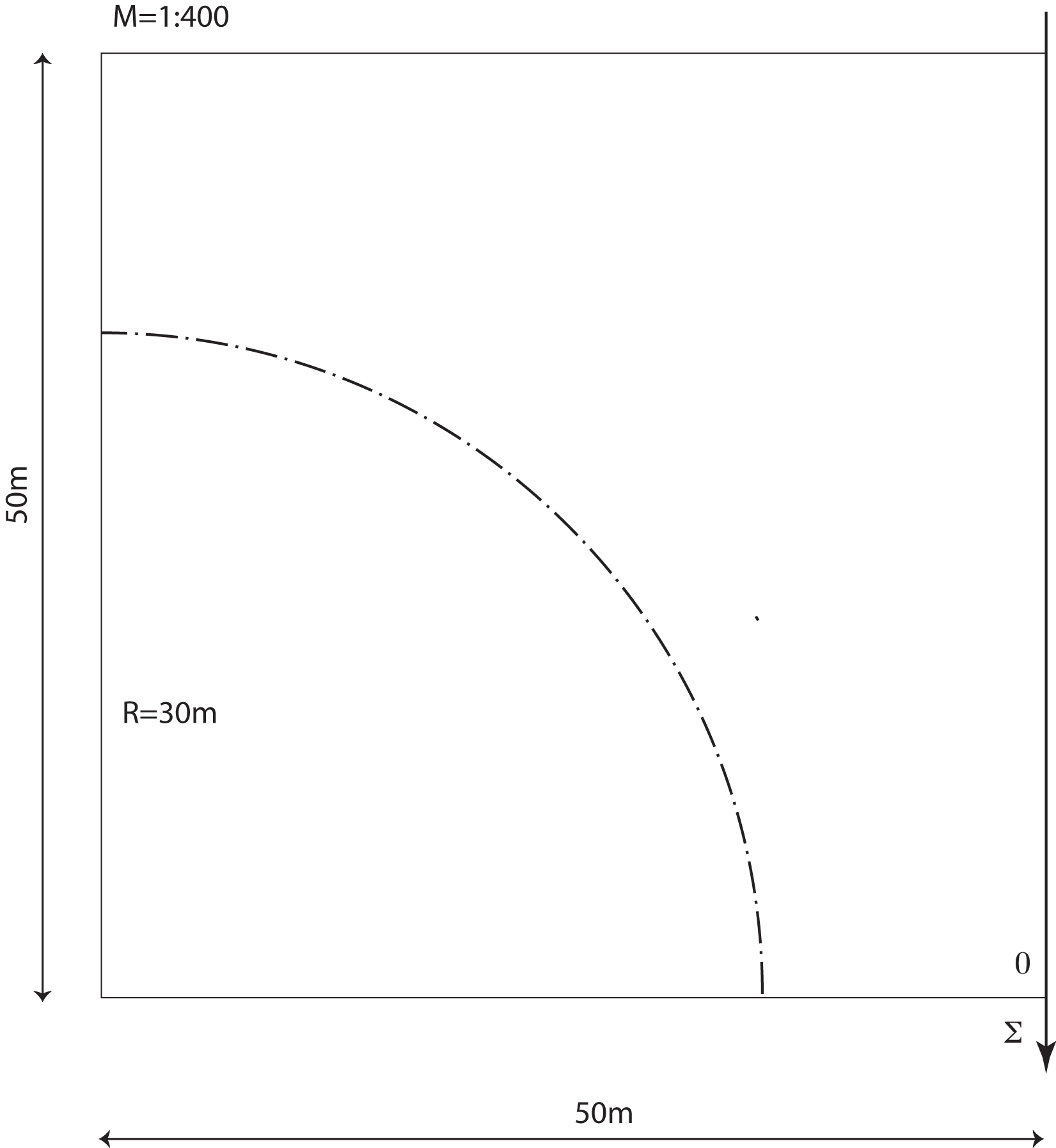1st solution - elevated projection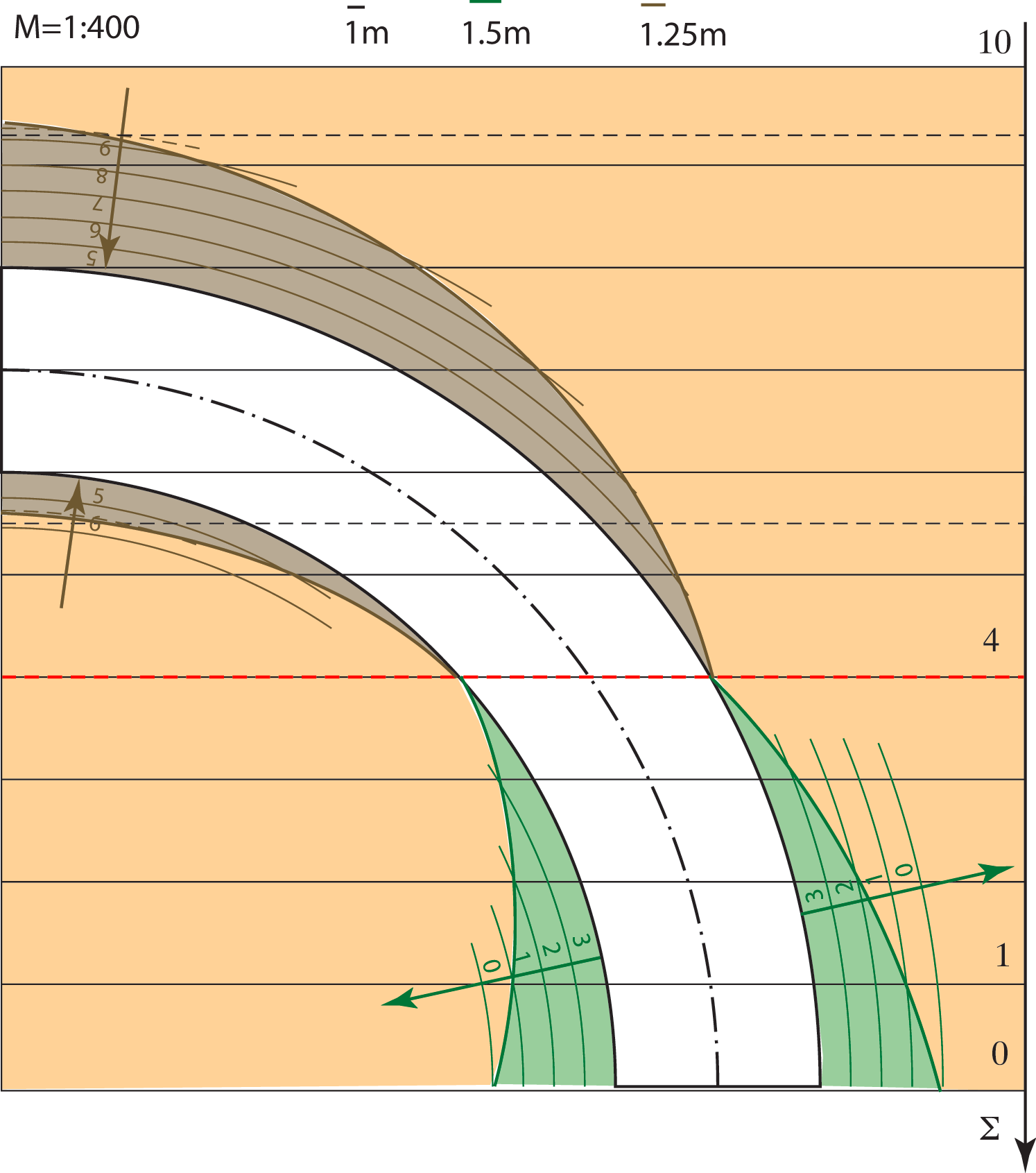2nd solution - program Rhinoideal terrain, subgrade and the neutral linefills and cuts

### A horizontal construction site with a straight horizontal road on a ideal terrain

EXERCISE 3: Let the plane $$\Sigma$$ be the ideal terrain with the slope 25%. On the template this plane is given with the scale of slope and the horizontal with the elevation 0.
The horizontal construction site has the elevation 16. The road has the width 10 and it is given with its axis on the template.
Solve the earthwork for building this road.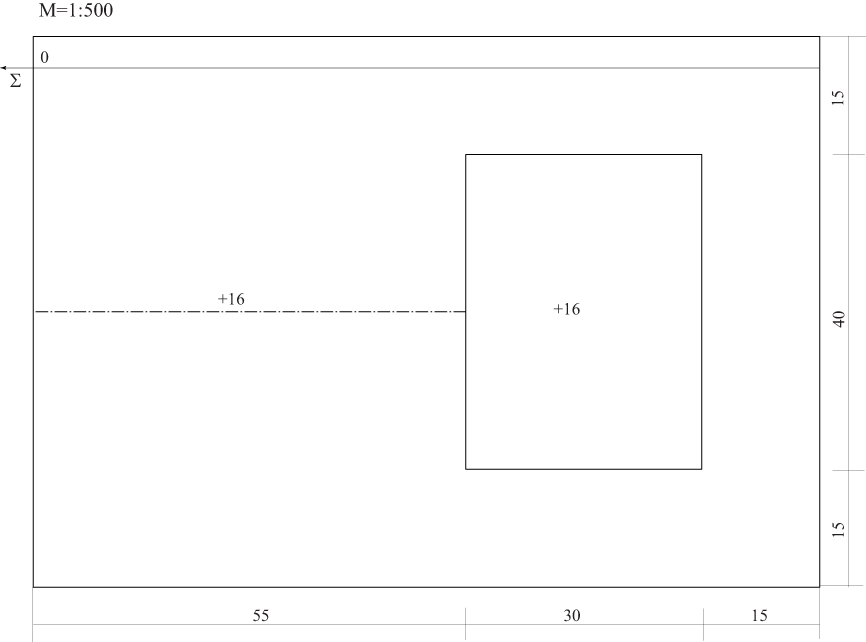1st solution - elevated projection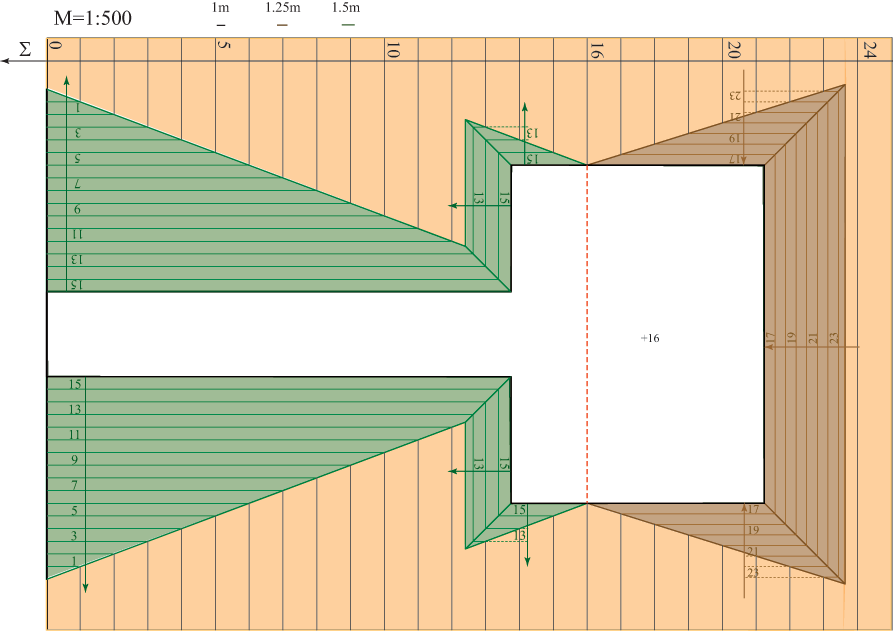Steps of the construction:
• Construct the horizontals of the plane $$\Sigma$$, $$i_\Sigma=4m$$.
• Construct the boundary line of the subgrade.
• Determine the neutral line.
• Find the scales of slope for 6 fills and construct the horizontals of those planes.
• Determine the boundary line between the ideal terrain and fills, i.e its intersection.
• Find the scales of slope for 3 cuts and construct the horizontals of those planes.
• Determine the boundary line between the ideal terrain and cuts, i.e its intersection.

2nd solution - program Rhinocuts (excavations)fills ( embankment)

### Horizontal road with a straight part and a part on a circular arc on a ideal terrain

EXERCISE 4: Let the plane $$\Sigma$$ be the ideal terrain with the slope 18%. On the template this plane is given with the scale of slope and the horizontal with the elevation 5.
The road has the width 12m and stretches along a straight part and a circular arc with the elevation 16. On the template the road is given with its axis.
Solve the earthwork for building this road.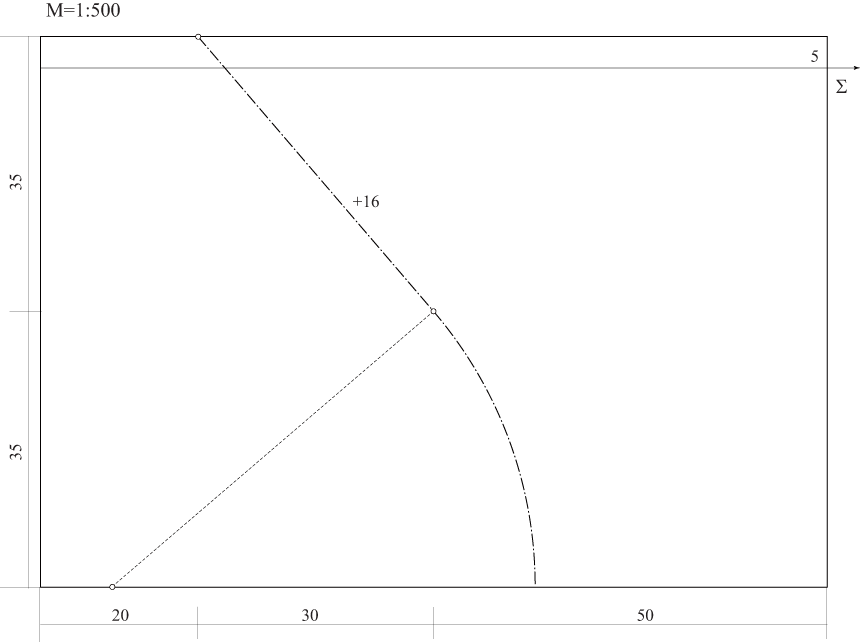1st solution - elevated projection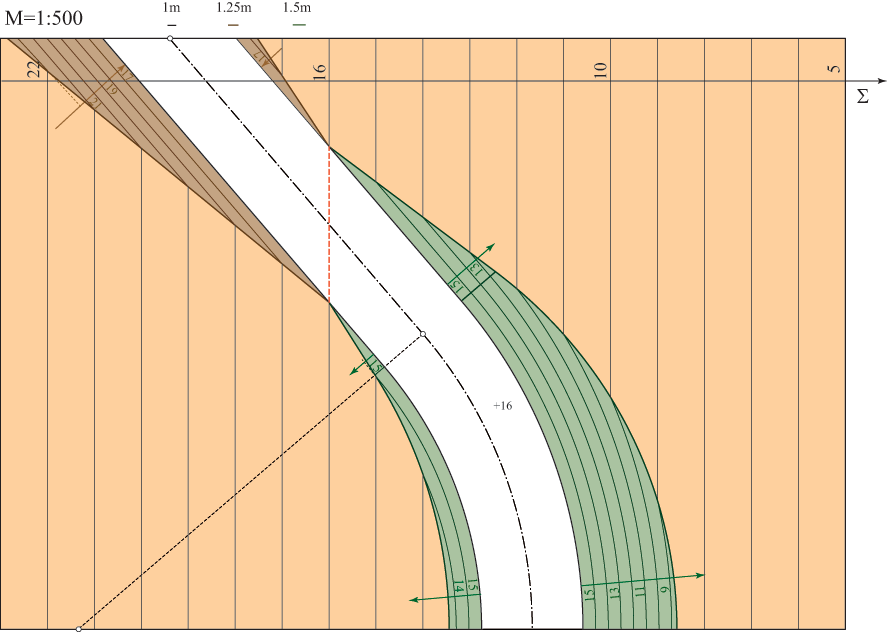2nd solution - program Rhinoideal terrain, subgrade and neutral linefills and cursfinal imagefinal image - horizontals

Created by Sonja Gorjanc and Ema Jurkin, translated by Helena Halas and Iva Kodrnja - 3DGeomTeh - Developing project of the University of Zagreb